# Fourier pseudo-spectral method

(diff) ← Older revision | Latest revision (diff) | Newer revision → (diff)

A type of trigonometric pseudo-spectral method (cf. Trigonometric pseudo-spectral methods) used to solve differential and integral equations. See also Chebyshev pseudo-spectral method.

The Fourier pseudo-spectral method is used for problems in which there is a natural periodicity. In multi-dimensional problems it should be used only in those directions with periodic boundary conditions. In non-periodic directions, Chebyshev methods, finite element or difference methods of high order should be used.

Suppose the equation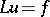is to be solved whereis a differential operator,is a given periodic function andis an unknown periodic function (period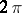). In the Fourier pseudo-spectral method, the solutionis approximated by a trigonometric polynomial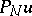that interpolatesat equally spaced points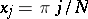,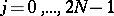.

The Lagrange interpolation polynomial (cf. also Lagrange interpolation formula) has the form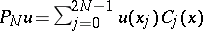, where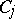is the Cardinal function, having the formAn equivalent way of defining the interpolating polynomial is by the discrete Fourier transform: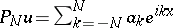, where the Fourier coefficients are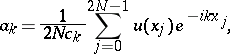In the Lagrange polynomial or "grid-point representation" , the problem can be written as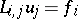, where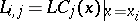The form of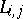can be found through differentiation of the Cardinal function: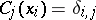,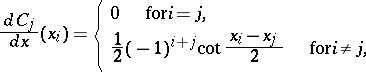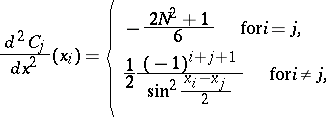to give a few. The derivative matrices are full and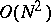operations are required for evaluation.

The problem can also be formulated using the spectral coefficient representation. Derivatives are found through differentiation of the discrete Fourier series, for example,and have an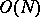operation count. If the operatoris non-linear or has non-constant coefficients multiplying the derivatives, evaluation ofis much more complicated.

The fast Fourier transform provides a way of switching between grid-point and spectral representations in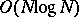operations, instead of theusing the definitions above. Derivatives can be accomplished in spectral space and multiplication of non-constant coefficients or evaluation of non-linear terms can be accomplished in grid point space — each withoperations.

Particularly for time-dependent problems in which boundary value problems must be solved at each time step, it is very important to minimize operation counts by taking full advantage of each representation and the fast transformation between representations.

As an example, consider the operator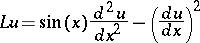for the time-dependent problem. Suppose at a particular time the spectral coefficients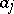,, are known. To compute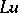, first the spectral coefficients of the first- and second-order derivatives are found:and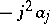. Then both of these arrays are transformed into their grid point representation using a fast Fourier transformation. Thencan be evaluated inoperations. The complete evaluation of the operator is done inoperations rather thanif done completely in one space. This property becomes even more critical for multi-dimensional problems; the operation counts areversus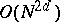for a-dimensional problem.

How to Cite This Entry:
Fourier pseudo-spectral method. Encyclopedia of Mathematics. URL: http://encyclopediaofmath.org/index.php?title=Fourier_pseudo-spectral_method&oldid=14397
This article was adapted from an original article by Richard B. Pelz (originator), which appeared in Encyclopedia of Mathematics - ISBN 1402006098. See original article